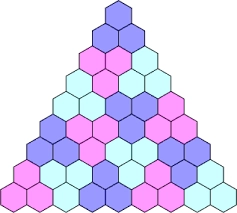# The sum 39

The sum of the first six terms of the arithmetic sequence is 72, and the second term is seven times the fifth term. Find the first term and the common difference.

a1 =  27
d =  -6

### Step-by-step explanation:Did you find an error or inaccuracy? Feel free to write us. Thank you!

Tips for related online calculators
Do you have a linear equation or system of equations and looking for its solution? Or do you have a quadratic equation?